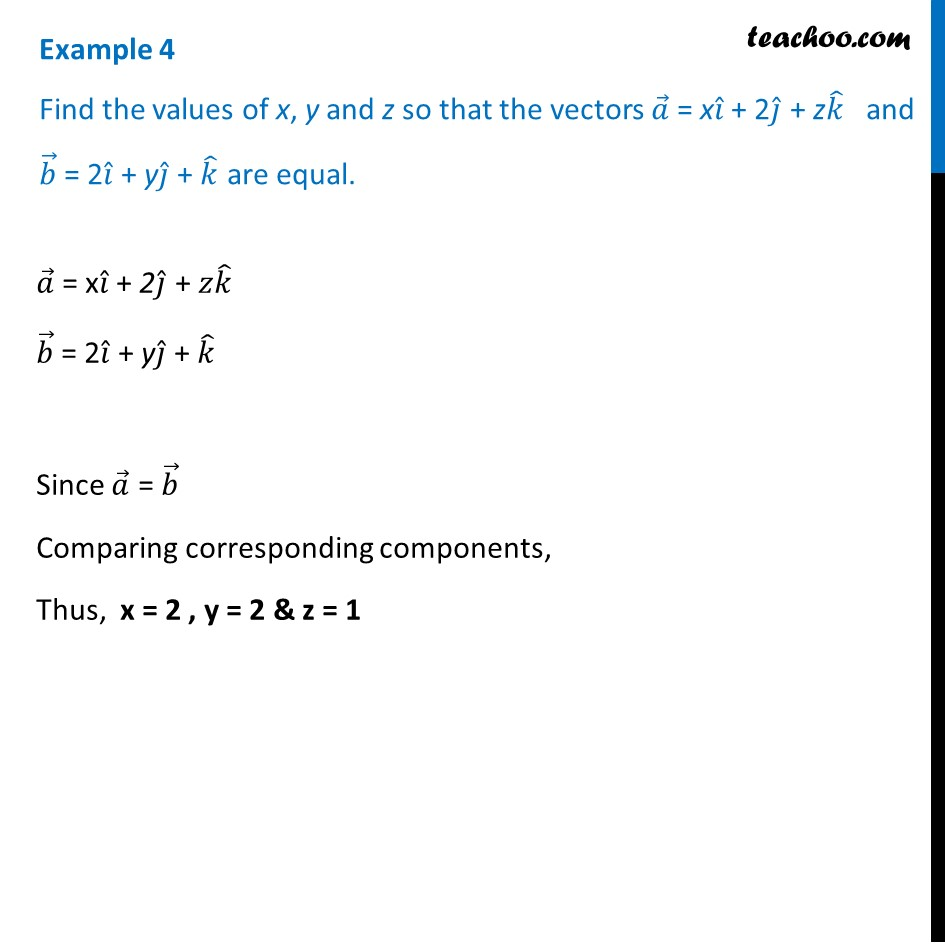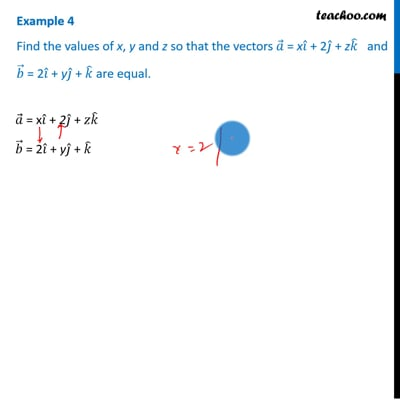Equal vectors

Chapter 10 Class 12 Vector Algebra
Concept wiseThis video is only available for Teachoo black users

Introducing your new favourite teacher - Teachoo Black, at only ₹83 per month

### Transcript

Examaple 4 Find the values of x, y and z so that the vectors 𝑎 ⃗ = x𝑖 ̂ + 2𝑗 ̂ + z𝑘 ̂ and 𝑏 ⃗ = 2𝑖 ̂ + y𝑗 ̂ + 𝑘 ̂ are equal. 𝑎 ⃗ = x𝑖 ̂ + 2𝑗 ̂ + 𝑧𝑘 ̂ 𝑏 ⃗ = 2𝑖 ̂ + y𝑗 ̂ + 𝑘 ̂ Since 𝑎 ⃗ = 𝑏 ⃗ Comparing corresponding components, Thus, x = 2 , y = 2 & z = 1 𝑎 ⃗ = x𝑖 ̂ + 2𝑗 ̂ + 𝑧𝑘 ̂ 𝑏 ⃗ = 2𝑖 ̂ + y𝑗 ̂ + 𝑘 ̂ Since 𝑎 ⃗ = 𝑏 ⃗ Comparing corresponding components, Thus, x = 2 , y = 2 & z = 1